# We Helped With This Mechanical Engineering Assignment: Have A Similar One?

SOLVEDCategory Engineering Mechanical Engineering Undergraduate Solved Mechanical Engineering Assignment Help

## Assignment DescriptionENG2005

Computational Module 1 - Matlab Primer

Assessed Problems

Clayton Campus

Malaysia Campus

Semester 1 and 2, 2017AustraliaMalaysiaSouth Africa         Italy         India       monash.edu/science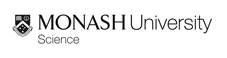SCHOOL OF MATHEMATICAL SCIENCES

ENG2005

Lab 1 - A Brief Matlab Primer

Assessed problems

The following assesed problems are based on the material covered in the notes ‘Lab 1 - A brief Matlab primer’. They are to be attempted after reading through those notes and are to be assessed in person by the demonstrator in your assigned laboratory class. Simply complete the problems (on your own!) and present the scripts to your demonstrator, showing how they work.

If you are having trouble completing any of the exercises or you are unsure of how the assessment for these modules works then feel free to ask the demonstrator for assistance!

Problem 1. In this course it will be sometimes necessary to integrate known functions over a given domain. Such integrals will often have no analytic solutions (or will be extremely difficult to solve analytically). We can numerically approximate such integrals with ease by writing a simple Matlab script.

The number resulting from evaluating the definite integral,is of course the area between the curve f(t) and the t-axis between t = a and t = b. The trapezoidal rule, for instance, simply breaks the area up into trapezoids which have simple areas that can be easily summed. In figure 1 for instance we see that the area between f(t) = sin(2πt) and the t-axis is approximated with 7 blocks (of area Ai) and 8 points (ti). The area of the i-th block, Ai is therefore calculated as,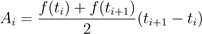.

If the points ti are evenly spaced and there are N t points, then summing up all of the areas generalizes to,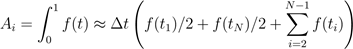,

where t = ti+1 ti. This is pretty simple to code up in a matlab script. Something like the following will get you an accurate result:

t = linspace(0,1,8); y = sin(2 ∗ pi t); School of Mathematical Sciences          Monash UniversityI = 0;

for i = 2 : length(t)

I = I + 0.5 ∗ (y(i) + y(i − 1)) ∗ (t(i) − t(i − 1)); end

Of course you know what the final integral should equal (given the function and the bounds over which it is being integrated) don’t you?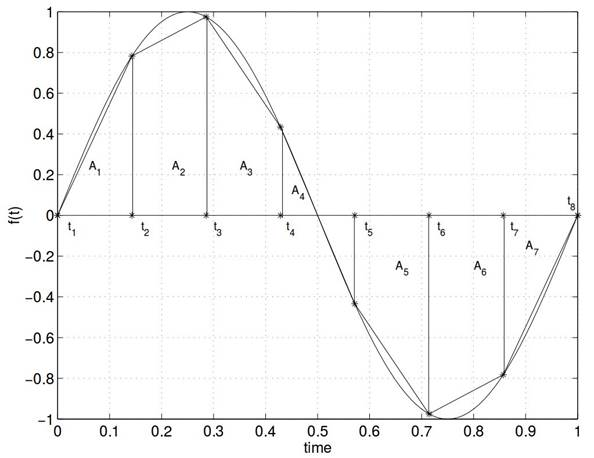Figure 1: The area under the curve sin(2πt) in the domain 0 ≤ t ≤ 1 is approximated by trapezoids of area Ai.

Create a script that uses the trapezoidal rule to integrate the following functions (separately) over 0 ≤ t ≤ 1: cos(t)et and |cos(2πt)| (you will have to use the ‘abs’ command for the second function). Try integrating with 2, 10 and 100 trapezoids. Of the two functions that you are integrating, which function is more sensitive to a wide (inaccurate) trapezoid size? Why is this?

Problem 2. Create a script which plots two functions on the same plot. The first function is,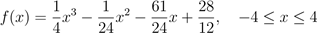.

Tuesday 14th February, 2017  3 School of Mathematical Sciences    Monash UniversityThe second function is a parametric curve,

x(t) = 2tcos(πt),       y(t) = 4sin(πt),       0 ≤ t ≤ 2.

You will have to create the t array and then determine the x and y arrays from that. Add labels, a legend and whatever fancy colours and line styles you wish.

Extend the above script to also plot (in a separate figure) the following multivariable function, g(x,y) = 7sin(πx)cos(3πy), −1 ≤ x,y ≤ 1,

whichever way you feel is best. Add labels and a title. Use the ‘subplot’ option to put another plot beside this one showing some contours of g. You may perhaps need to use some combination of the ‘meshgrid’, ’contour’ and ‘surf’ (or ‘surfc’) functions.

Problem 3. Create a Matlab function that takes an integer N as input and calculates and then returns the fibonacci sequence up to the N-th term. You should be able to call this new function from the terminal, so make sure that it is saved in your current working directory and ensure that it’s name doesnt conflict with any pre-existing Matlab functions.

Tuesday 14th February, 2017                                                                                                                                 4

Is it free to get my assignment evaluated?

Yes. No hidden fees. You pay for the solution only, and all the explanations about how to run it are included in the price. It takes up to 24 hours to get a quote from an expert. In some cases, we can help you faster if an expert is available, but you should always order in advance to avoid the risks. You can place a new order here.

How much does it cost?

The cost depends on many factors: how far away the deadline is, how hard/big the task is, if it is code only or a report, etc. We try to give rough estimates here, but it is just for orientation (in USD):

 Regular homework \$20 - \$150 Advanced homework \$100 - \$300 Group project or a report \$200 - \$500 Mid-term or final project \$200 - \$800 Live exam help \$100 - \$300 Full thesis \$1000 - \$3000

How do I pay?

Credit card or PayPal. You don't need to create/have a Payal account in order to pay by a credit card. Paypal offers you "buyer's protection" in case of any issues.

Why do I need to pay in advance?

We have no way to request money after we send you the solution. PayPal works as a middleman, which protects you in case of any disputes, so you should feel safe paying using PayPal.

Do you do essays?

No, unless it is a data analysis essay or report. This is because essays are very personal and it is easy to see when they are written by another person. This is not the case with math and programming.

Why there are no discounts?

It is because we don't want to lie - in such services no discount can be set in advance because we set the price knowing that there is a discount. For example, if we wanted to ask for \$100, we could tell that the price is \$200 and because you are special, we can do a 50% discount. It is the way all scam websites operate. We set honest prices instead, so there is no need for fake discounts.

Do you do live tutoring?

No, it is simply not how we operate. How often do you meet a great programmer who is also a great speaker? Rarely. It is why we encourage our experts to write down explanations instead of having a live call. It is often enough to get you started - analyzing and running the solutions is a big part of learning.

What happens if I am not satisfied with the solution?

Another expert will review the task, and if your claim is reasonable - we refund the payment and often block the freelancer from our platform. Because we are so harsh with our experts - the ones working with us are very trustworthy to deliver high-quality assignment solutions on time.

Customer Feedback

"Thanks for explanations after the assignment was already completed... Emily is such a nice tutor! "

Order #13073